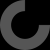Report as inappropriate

I've had an issue with using merge=true along with exRot rotations, where the end points didn't match up correctly. As a quick hack, I've disabled the spreadError code via an added optional parameter to get it working as expected. Here's the patch:

--- path_extrude.scad.orig   2019-03-27 13:14:41.932931102 -0700
+++ path_extrude.scad     2019-03-27 13:13:27.059513667 -0700
@@ -182,7 +182,7 @@
myPointsChunk = [ for(t = [45:(360/8):136]) ((t==90)?1.5:1.9) * [cos(t+ofs1),sin(t+ofs1)]];
//myPoints = [ for(t = [0:(360/8):359]) 2 * [cos(t+45),sin(t+45)]];

-module path_extrude(exPath = myPath, exShape = myPoints, exRots = , merge=false){
+module path_extrude(exPath = myPath, exShape = myPoints, exRots = , merge=false, spreadError=true){
if((exShape == undef) || (exPath == undef)){
echo("Path or shape not defined");
} else {
@@ -200,7 +200,7 @@
myRotate([0,0,-rawPreRots[len(rawPreRots)-1]], c3D(exShape)));
pt0 = project(p1=t0, c1=pm1, n1=rPlanes[len(rPlanes)-1]);
lfAng = -getNPAngle(p1 = pt0, c1 = pm1, n1=rPlanes[len(rPlanes)-2], p2=tm1);
-        preRots = (merge) ? spreadError(rawPreRots, -lfAng) : rawPreRots;
+        preRots = (merge && spreadError) ? spreadError(rawPreRots, -lfAng) : rawPreRots;
polyPoints = flatten(makePolyPoints(polyPath=exPath, polyForm=exShape,
polyAngles=preRots, polyNormals=rPlanes, merge=merge));

If you render the attached file with the default spreadError=true, the start and end of the path are unexpectedly connected with straight segments due to the last slice being rotated to match the start slice. With the option disabled, I get the expected smooth join. See the attached pictures for comparison.

Thanks for making this library! This is very useful.

Edit: fixed the diff, I had gotten the patch order backwards.